# Voltmeter range

We have a voltmeter which in the original set measures voltage to 10V. Calculate the size of the ballast resistor for this voltmeter, if we want to measure the voltage up to 50V. Voltmeter's internal resistance is 2 kiloohm/Volt.

Result

R2 =  80000 Ω

#### Solution:

$U_{1}=10 \ \text{V} \ \\ U_{2}=50 \ \text{V} \ \\ \ \\ R_{1}=2 \cdot \ 1000 \cdot \ U_{1}=2 \cdot \ 1000 \cdot \ 10=20000 \ Ω \ \\ U_{3}=U_{2}-U_{1}=50-10=40 \ \text{V} \ \\ \ \\ I=\dfrac{ U_{1} }{ R_{1} }=\dfrac{ 10 }{ 20000 }=\dfrac{ 1 }{ 2000 }=0.0005 \ \text{A} \ \\ U_{3}=I \cdot \ R_{2} \ \\ \ \\ R_{2}=U_{3}/I=40/0.0005=80000=80000 \ Ω$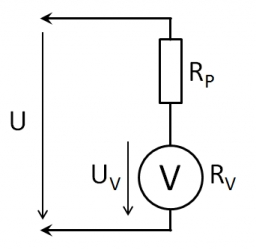Our examples were largely sent or created by pupils and students themselves. Therefore, we would be pleased if you could send us any errors you found, spelling mistakes, or rephasing the example. Thank you!

Leave us a comment of this math problem and its solution (i.e. if it is still somewhat unclear...):Be the first to comment!## Next similar math problems:

1. Resistance of the resistorThe resistor terminals have a voltage of 20 V and a current of 5 mA is passed through. What is the resistance of the resistor?
2. Filament of bulbThe filament of bulb has a 1 ohm resistivity and is connected to a voltage 220 V. How much electric charge will pass through the fiber when the electric current passes for 10 seconds?
3. Coil as a girl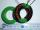The electrical resistance of the copper wire coil is 2.0 ohms. What current runs through the coil when the voltage between the terminals is 3.0 V?
4. Closed circuitIn a closed circuit, there is a voltage source with U1 = 12 V and with an internal resistance R1 = 0.2 Ω. The external resistance is R2 = 19.8 Ω. Determine the electric current and terminal voltage.
5. Cooker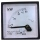A current of 2A passes through the immersion cooker at a voltage of 230V. What work do the electric field forces in 2 minutes?
6. Fog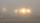The car started in fog at speed 30 km/h. After a 12-minute drive, the fog dissipated and the driver drove next 12 minutes distance 17 km. On the last 17 km long again the driving conditions deteriorated and the driver drove the speed of 51 km/h. a) Calc
7. Copper Cu wireCopper wire with a diameter of 1 mm and a weight of 350 g is wound on a spool. Calculate its length if the copper density is p = 8.9 g/cm cubic.
8. The shooter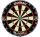The shooter heard the impact of the bullet on the target in one second after the shot. The bullet was moving at an average speed of 500 m/s. Calculate with speed of sound of 340 m/s. Determine the distance of the target.
9. TransformerSolve the textbook problems - transformer: a) N1 = 40, N2 = 80, U2 = 80 V, U1 =? b) N1 = 400, U1 = 200 V, U2 = 50 V, N2 =?
10. ResistanceDetermine the resistance of the bulb with current 200 mA and is in regular lamp (230V).
11. The coal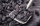The coal stock would be enough to heat a larger room for 12 weeks, a smaller one for 18 weeks. It was heated for four weeks in both rooms, then only in a smaller one. How long was the coal stock enough?
12. The copper wireThe copper wire bundle with a diameter of 2.8mm has a weight of 5kg. How many meters of wire is bundled if 1m3 of copper weighs 8930kg?
13. Aircraft angines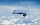The two engines of the aircraft are enough to supply the fuel for five hours of operation. However, one of the engines has a malfunction and thus consumes one-third more fuel. How long can the plane be in the air before it runs out of fuel? After an hour
14. Water current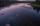John swims upstream. After a while, he passes the bottle, from that moment he floats for 20 minutes in the same direction. He then turns around and swims back, and from the first meeting with the bottle, he sails 2 kilometers before he reaches the bottle.
15. Cheetah vs antelope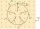When the cheetah began chasing the antelope, the distance between them was 120 meters. Although the antelope was running at 72km/h, the cheetah caught up with it in 12 seconds. What speed was the cheetah running?
16. Cyclists and walkersA group of tourists started at 8:00 at speed 4 km/h walk. At half-past ten, another group started on a bike and caught up with a group of tourists at 10:50. What was the average speed of cyclists?
17. Where and when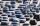The truck left Kremnica at 11:00 h at a speed of 60km/h. At 12:30 h, the passenger car started at an average speed of 80km/h. How many kilometers from Kremnica will the passenger car reach truck, and when?